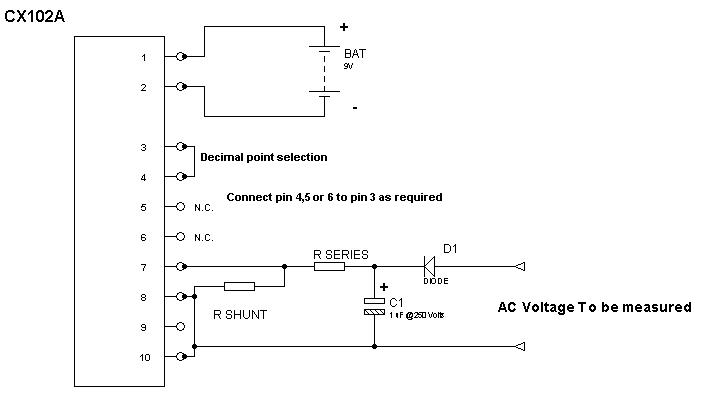# Use a Digital Panel Meter (DPM) to Measure AC Voltage

Digital Panel Meters (DPMs) are strictly DC meters due to the digital circuitry used. Oftentimes it is desired to utilize a DPM to measure AC voltages to take advantage of the improved accuracy and readability of a DPM. This application note will describe the method to accurately display AC voltage values on a DPM. The CX102A Digital Panel Meter from Circuit Specialists is ideal for this application, as it is designed to use in a system that has the measured signal isolated from the power supply voltage. The application is for a 0-120 volt AC meter powered by an external 9 volt battery. This application could also be powered by a “wall-wart” type of AC adapter if desired. Like all Digital Panel Meters, the full scale range of this DPM is 200 mv full-scale.

## How to Measure AC Voltages with a DPM

To use the DPM to measure AC voltages, the AC voltage must be converted to DC by a rectifier diode. The output from the rectifier diode will be a “pulsed” DC voltage and may produce undesired fluctuations in the reading on the DPM, so we will add a small filter capacitor across the rectified output voltage. The voltage rating of this capacitor and the rectifier diode must be high enough to handle the voltage levels present in the circuit.

To be safe, we will use a rectifier diode and capacitor rated at 250 volts or higher. Since we are using a single rectifier diode to convert the AC into DC, we will have to choose our divider resistors appropriately to compensate for the effects of the half wave rectifier operation. Since the AC power line voltage is rectified by the series diode, the voltage applied to our DPM is the peak value of the 120 volt line voltage which is 120 V multiplied by 1.414 . Vout = Vin X (1.414) = 120 X (1.414) = 169 V (DC) This is the actual DC voltage applied to our voltage divider resistors, so we will use the voltage divider formula to determine the required resistors to produce the correct reading on the DPM.

To keep the calculated values within a range that is readily obtainable, we will use 10 Meg ohms as the maximum series resistor value. We can then calculate the shunt resistor for our voltage divider network using the voltage divider equation Vout/Vin=Rshunt/(Rseries + Shunt) rearranging we can solve for the required Use Rseries = 10 Meg Ohms For 0-120 Volts, Rshunt = 7.2 K ohms (use 7.5 K ohm) We will also need to connect pin 3 to pin 4 (for proper decimal point display).

Note that the voltage value displayed on the meter can be fine-tuned by adjusting the trimmer potentiometer on the back of the DPM. This application note has shown how a Digital Panel Meter may be used to measure and display AC voltage values. The connection diagram is shown below. Note that pins 8 & 10 are shorted together and connected to the Neutral connection of the AC voltage that is being measured. Rshunt will be connected across pins 7 & 8 and Rseries will have one end connected to pin 7 and the other end to the voltage that is being measured.Comments must be approved before appearing